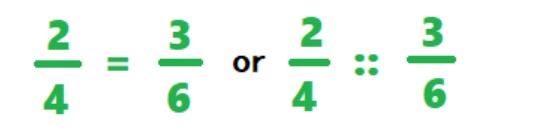# Proportion Calculator

Enter an unknown variable (x) and 3 values in the input boxes below to find the proportion between two ratios using proportions calculator.

Looking for Ratio calculator?

=

Give Us Feedback

Proportion calculator is an online tool that solves two fractions for the variable x. It evaluates the equality between two fractions with cross multiplication.

## What are proportions?

Proportion is a statement which tells us that two ratios are equal. The symbol of proportion is ‘::’ and ‘=’.

For example, two ratios are in proportion if they are equal to each other.## How to solve proportions?

Solving proportions is easier than you think. Let’s solve a proportion with an example.

Example:

If ratio of pizzas to burgers is 3/5 in a party, how many of burgers will be there if there are total of 15 burgers.

Solution:

Step 1: Construct a proportion using the given values and x.

3/5 = x/15

Step 2: Apply cross multiplication to the above equation.

5x = 3 × 15

x = 45/5

x = 9

So, there will be 9 pizzas with 15 burgers.

Use proportion solver above to solve fractions.

### Math Tools